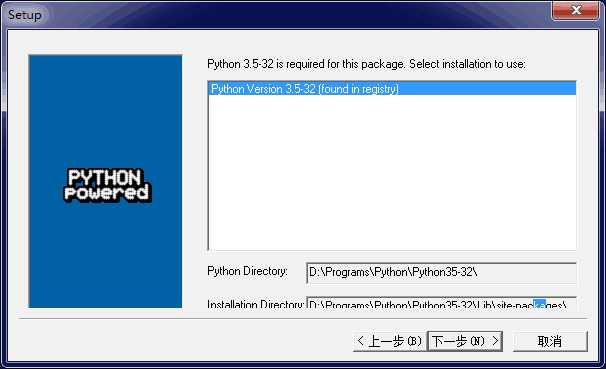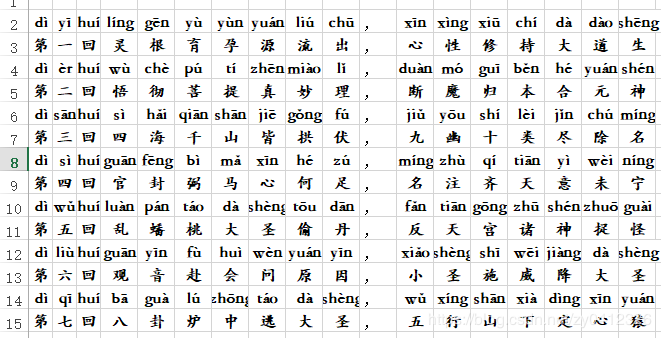# 基于Python第三方插件实现西游记章节标注汉语拼音的方法_python

MySQLdbMySQLdb是 Python 连接 MySQL 最流行的一个驱动，很多框架都也是基于此库进行开发，遗憾的是它只支持 Python2.x，它是基于C开发的库，和Windows 平台的兼容性不友好，现在基本不推荐使用，取代的是它的衍生版本。mysqlclient由于 MySQLdb 年久失修，后来出现了它的 Fork 版本 mysqlclient，完全兼容 MySQLdb，同时支持 Python3.x，是 Django ORM的依赖工具，如果你想使用原生 SQL 来操作数据库，那么推荐此驱动。PyMySQLPyMySQL是纯 Python 实现的驱动，速度上比不上 MySQLdb，最大的特点可能就是它的安装方式没那么繁琐，同时也兼容 MySQLdb。SQLAlchemySQLAlchemy是一种既支持原生 SQL，又支持 ORM 的工具，它非常接近 Java 中的 Hibernate 框架。有关Python访问MySQL数据库全部内容的学习，我都是通过黑马程序员的视频学的。看完之后立马决定去培训了。你可以搜搜，都是免费资源。黑马视频库里面搜索一下，找不到的话官网弹出来的对话框问一下就不会迷路了www.zgxue.com防采集请勿采集本网。

起因很单纯，就是给我1年级小豆包的女儿标注三国和西游章节的汉语拼音，我女儿每天都朗读 ，结果有很多字不认识，我爱人居然让我给标记不认识的完了手动注音......我勒个去......身为程序员的我怎么能忘记用程序实现呢，特别是咱也会点Python万能语言。哈哈！列举一下使用的技术。# 带声调的(默认)def yinjie(word): sentens = "".join(word.split()) print(sentens) s = '' # heteronym=True开启多音字 for i in pypinyin.pinyin(word, heteronym=False): s = s + ''.join(i) + " " return s

['dì', '第'], ['yī', '一'], ['bǎi', '百'], ['huí', '回']

[['dì', '第'], ['yī', '一'], ['bǎi', '百'], ['huí', '回']], [['jìng', '径'], ['huí', '回'], ['dōng', '东'], ['tǔ', '土']], [['wǔ', '五'], ['shèng', '圣'], ['chéng', '成'], ['zhēn', '真']]import pypinyinfrom openpyxl import Workbookfrom openpyxl.drawing.text import Fontfrom openpyxl.styles import Font, colors, Alignmentfrom pulgin.Tools import Toolsclass HanZhiAddPinYin: def __init__(self): pass def signWord(self,word): pinyicontent = pypinyin.pinyin(word, heteronym=False) word_pinyin = [pinyicontent, word] return word_pinyin def sentences(self,keyWords): listsentense = [] for duanyu in keyWords.split(): print(duanyu) duanyu_list = [] for word in duanyu: duanyu_list.append(self.signWord(word)) listsentense.append(duanyu_list) print( listsentense ) return listsentense def articles(self,txt_file_path): article = [] encoding = Tools.get_file_encoding(txt_file_path) f = open(txt_file_path, "r", encoding=encoding, errors='ignore') # 返回一个文件对象 line = f.readline().strip() # 调用文件的 readline()方法 index = 1 while line: article.append(self.sentences(line)) line = f.readline() index = index + 1 f.close() return article def builder_execl(self,word_title, save_path, article): """ 构建execl文件 :param word_title: sheet页面的名词 :param save_path: execl保存路径 :param article: 文章内容集合 :return: """ wb = Workbook() ws = wb.active ws.title = word_title ws.sheet_properties.tabColor = "1072BA" # 设置背景 xl_sheet = wb.get_sheet_by_name(word_title) execl_cell_width = 4.6 for sentences in article: column_index = 1 # sentences 2行数据 # 获取行数 pinyin_row = xl_sheet.max_row + 1 # 拼音所在的行 hanzi_row = pinyin_row + 1 # 汉字所在的行 sentences_index = 0 for duanyu in sentences: # ['dì', '第'], ['yī', '一'], ['huí', '回'] for sign_word in duanyu: # ['dì', '第'] # region 设置样式 # 设置样式 execl_cell_font = Font(name='华文楷体', size=12, italic=False, color=colors.BLACK, bold=True) execl_pinyin_row = xl_sheet.cell(row=pinyin_row, column=column_index) execl_hanzi_row = xl_sheet.cell(row=hanzi_row, column=column_index) execl_pinyin_row.alignment = Alignment(horizontal='center', vertical='center') execl_hanzi_row.alignment = Alignment(horizontal='center', vertical='center') execl_pinyin_row.font = execl_cell_font execl_hanzi_row.font = execl_cell_font xl_sheet.column_dimensions['A'].width = 3 xl_sheet.column_dimensions['B'].width = 3 xl_sheet.column_dimensions['C'].width = 3 xl_sheet.column_dimensions['D'].width = execl_cell_width xl_sheet.column_dimensions['E'].width = execl_cell_width xl_sheet.column_dimensions['F'].width = execl_cell_width xl_sheet.column_dimensions['H'].width = execl_cell_width xl_sheet.column_dimensions['I'].width = execl_cell_width xl_sheet.column_dimensions['G'].width = execl_cell_width xl_sheet.column_dimensions['J'].width = execl_cell_width xl_sheet.column_dimensions['K'].width = execl_cell_width xl_sheet.column_dimensions['L'].width = execl_cell_width xl_sheet.column_dimensions['M'].width = execl_cell_width xl_sheet.column_dimensions['N'].width = execl_cell_width xl_sheet.column_dimensions['O'].width = execl_cell_width xl_sheet.column_dimensions['P'].width = execl_cell_width xl_sheet.column_dimensions['Q'].width = execl_cell_width xl_sheet.column_dimensions['R'].width = execl_cell_width xl_sheet.column_dimensions['S'].width = execl_cell_width xl_sheet.column_dimensions['T'].width = execl_cell_width xl_sheet.column_dimensions['U'].width = execl_cell_width xl_sheet.column_dimensions['V'].width = execl_cell_width xl_sheet.column_dimensions['W'].width = execl_cell_width # endregion xl_sheet.cell(row=pinyin_row, column=column_index, value=sign_word) xl_sheet.cell(row=hanzi_row, column=column_index, value=sign_word) # 0 第一百回 1 径回东土 2 五圣成真 # print(sentences_index) # print(len(duanyu) + len(sentences)) # print(column_index) if sentences_index == 1 and len(duanyu) + len(sentences) == column_index:#坑人的and xl_sheet.cell(row=pinyin_row, column=column_index + 1, value="，") xl_sheet.cell(row=hanzi_row, column=column_index + 1, value="，") column_index = column_index + 1 # 遇到断句多增加一列向后 column_index = column_index + 1 # 列向后 sentences_index = sentences_index + 1 # 三个短语计数器 wb.save(save_path)

• 本文相关：
• python模块汇总(常用第三方库)
• python实现关闭第三方窗口的方法
• python第三方库face_recognition在windows上的安装过程
• python使用到第三方库pymupdf图片与pdf相互转换
• 利用python实现汉字转拼音的2种方法
• python获取一组汉字拼音首字母的方法
• python实现将汉字转换成汉语拼音的库
• python 返回汉字的汉语拼音
• python从网络读取图片并直接进行处理的方法
• tensorflow打印输出tensor的值
• python中for循环详解
• python+pandas分析nginx日志的实例
• 如何使用python 打印各种三角形
• python itsdangerous模块的具体使用方法
• python内建数据结构详解
• 快速了解python中的装饰器
• python通过for循环理解迭代器和生成器实例详解
• Python 常用的标准库以及第三方库有哪些
• Python 访问 MySQL 数据库可以使用哪些第三方实现？
• Python几种主流框架比较
• 如何利用python的第三方接口进行二次开发
• 什么是目前比较常用的Python扩展库管理工具
• 学会python可以干什么
• python 适合做什么开发
• 如何用Python实现与知乎相关的某些小脚本+某些福利的获取
• 为什么人工智能用 Python
• python是什么语言
• 免责声明 - 关于我们 - 联系我们 - 广告联系 - 友情链接 - 帮助中心 - 频道导航